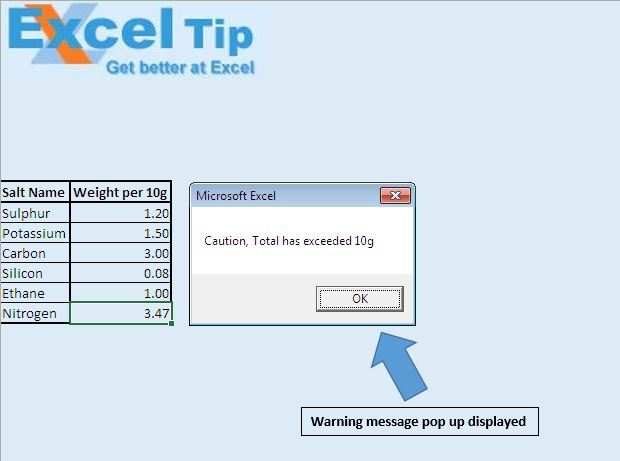# Display message if column total exceeds using VBA in Microsoft Excel

In this article, we will use change event of worksheets to display a warning message when the column total exceeds total value.

Raw data consists of salt composition of a medicine. It comprises of salt name and it's weight per 10 grams.Total of all the salt ingredients is equal to 10 grams. When we change the weight of ethane salt from 0.75 to 1, a warning message will pop-up.Logic explanation

In this article, we have used change event of worksheet to generate warning message. Change event checks the total of 2nd column, which should not exceed 10.

Code explanation

If Target.Column = 2 Then

The above code is used to restrict change event to trigger only when value in the 2nd column is changed.

WorksheetFunction.Sum(Columns(2))

The above code is used to get sum of all the values in column 2.

```Option Explicit

Private Sub Worksheet_Change(ByVal Target As Range)

'Checking whether value is changed in second column
If Target.Column = 2 Then

'Checking whether sum of values in second column is greater than 10
If WorksheetFunction.Sum(Columns(2)) > 10 Then
MsgBox "Caution, Total has exceeded 10g"
End If

End If

End Sub
```